# Dirichlet Integral

(redirected from Dirichlet Integrals)
The following article is from The Great Soviet Encyclopedia (1979). It might be outdated or ideologically biased.

## Dirichlet Integral

(named for P. G. L. Dirichlet), the name of several types of integrals.

(1) The integral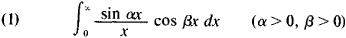This Dirichlet integral is also called the Dirichlet discontinous factor and is equal to π/2 for β < a, to β/4 for β = α, and to 0 for β > α. Thus, Dirichlet integral (1) is a discontinous function of parameters α and β. Dirichlet used the integral (1) in his studies of the attraction of ellipsoids. However, this integral appeared earlier in the works of J. Fourier, S. Poisson, and A. M. Legendre.

(2) The integral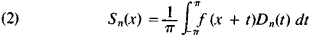where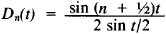is the so-called Dirichlet kernel. This Dirichlet integral is equal to the nth partial sum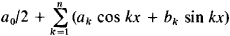of a Fourier series of the function f(x). Formula (2) is one of the most important formulas in the theory of Fourier series; in particular, it enabled Dirichlet to show that the Fourier series of a function with a finite number of maxima and minima converges at every point.

(3) The integral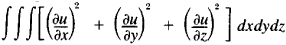The Great Soviet Encyclopedia, 3rd Edition (1970-1979). © 2010 The Gale Group, Inc. All rights reserved.
References in periodicals archive ?
The modulus of a quadrilateral and Dirichlet integrals. One can express the modulus of a quadrilateral (D; [z.sub.1], [z.sub.2], [z.sub.3], [z.sub.4]) in terms of the solution of the Dirichlet-Neumann problem as follows.
The modulus of a ring domain and Dirichlet integrals. Let E and F be two disjoint compact sets in the extended complex plane C[infinity].
in Section 2, before we present our main results, we first establish a sufficient condition for univalence for functions in A(p) and we feel that it will be useful to present the absolute estimates for the Dirichlet integrals [DELTA](r, f) and [DELTA](r, f /z) for f [member of] [SIGMA](p) and 0 < r < p.
WIRTHS, Integral means and Dirichlet integral for analytic functions, Math.
Japanese mathematicians Fukushima (Osaka U.), Yoichi Oshima (Kumamato U.), and Masayoshi Takeda (Tohoku U.) begin with an introductory and comprehensive account of the theory of (symmetric) Dirichlet forms, which they explain as an axiomatic extension of the classical Dirichlet integrals in the direction of Markovian semigroups.
Sobel, Uppuluri, and Frankowski  tabulated the incomplete Dirichlet integrals of Type 2:
Frankowski, Dirichlet Integrals of Type 2 and Their Applications, in R.
Site: Follow: Share:
Open / Close